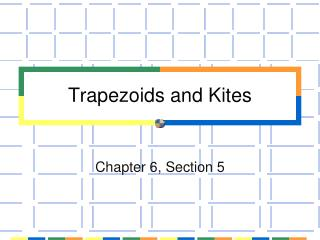# Trapezoids and Kites - PowerPoint PPT PresentationDownload PresentationTrapezoids and Kites

Trapezoids and KitesDownload Presentation## Trapezoids and Kites

- - - - - - - - - - - - - - - - - - - - - - - - - - - E N D - - - - - - - - - - - - - - - - - - - - - - - - - - -
##### Presentation Transcript

1. Trapezoids and Kites Chapter 6, Section 5

2. Essential Questions • How do I use properties of trapezoids? • How do I use properties of kites?

3. base leg leg base Vocabulary • Trapezoid – a quadrilateral with exactly one pair of parallel sides. • A trapezoid has two pairs of base angles. In this example the base angles are A & B and C & D

4. Base Angles Trapezoid Theorem • If a trapezoid is isosceles, then each pair of base angles is congruent.  A  B,  C  D

5. Base Angles Trapezoid Converse • If a trapezoid has a pair of congruent base angles, then it is an isosceles trapezoid. ABCD is an isosceles trapezoid

6. Diagonals of a Trapezoid Theorem • A trapezoid is isosceles if and only if its diagonals are congruent.

7. Example 1 • PQRS is an isosceles trapezoid. Find m P, m Q and mR. m R = 50 since base angles are congruent mP = 130 and mQ = 130 (consecutive angles of parallel lines cut by a transversal are )

8. Definition • Midsegment of a trapezoid – the segment that connects the midpoints of the legs.

9. Midsegment Theorem for Trapezoids • The midsegment of a trapezoid is parallel to each base and its length is one half the sum of the lengths of the bases.

10. Definition • Kite – a quadrilateral that has two pairs of consecutive congruent sides, but opposite sides are not congruent.

11. Theorem: Perpendicular Diagonals of a Kite • If a quadrilateral is a kite, then its diagonals are perpendicular.

12. A C, B D Theorem: Opposite Angles of a Kite • If a quadrilateral is a kite, then exactly one pair of opposite angles are congruent

13. Example 2 • Find the side lengths of the kite.

14. Example 2 Continued We can use the Pythagorean Theorem to find the side lengths. 122 + 202 = (WX)2 144 + 400 = (WX)2 544 = (WX)2 122 + 122 = (XY)2 144 + 144 = (XY)2 288 = (XY)2

15. Example 3 • Find mG and mJ. Since GHJK is a kite G J So 2(mG) + 132 + 60 = 360 2(mG) =168 mG = 84 and mJ = 84

16. Try This! • RSTU is a kite. Find mR, mS and mT. x +30 + 125 + 125 + x = 360 2x + 280 = 360 2x = 80 x = 40 So mR = 70, mT = 40 and mS = 125